# June 3rd Electronics TV's & Laptops

### Auction Details

Electronics TV's & Laptops
Choose Your Layout >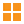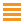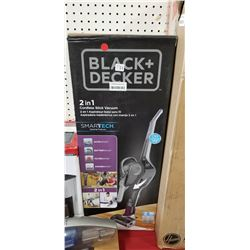51 - Shelf One
CDC Auctions
Shelf One
Bidding Has Concluded

Sold to d*******M for (32.50 + 4.23) x 1 = 36.73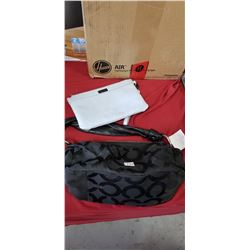52 - Shelf Two
CDC Auctions
Shelf Two
Bidding Has Concluded

Sold to f***r for (15.00 + 1.95) x 1 = 16.95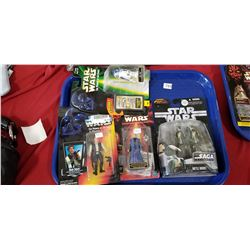53 - Shelf One
CDC Auctions
Shelf One
Bidding Has Concluded

Sold to r********r for (12.50 + 1.63) x 1 = 14.13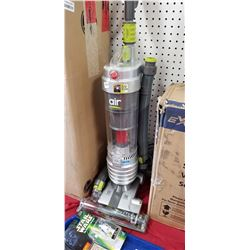54 - Shelf One
CDC Auctions
Shelf One
Bidding Has Concluded

Sold to f***r for (32.50 + 4.23) x 1 = 36.73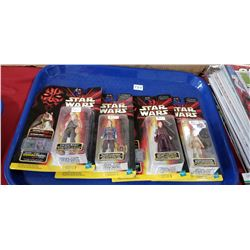55 - Shelf One
CDC Auctions
Shelf One
Bidding Has Concluded

Sold to r********r for (12.50 + 1.63) x 1 = 14.1357 - Shelf One
CDC Auctions
Shelf One
Bidding Has Concluded

Sold to f***r for (10.00 + 1.30) x 1 = 11.30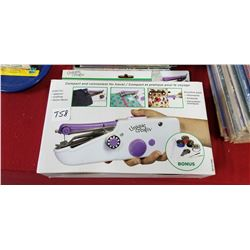58 - Shelf One
CDC Auctions
Shelf One
Bidding Has Concluded

Sold to f***r for (10.00 + 1.30) x 1 = 11.30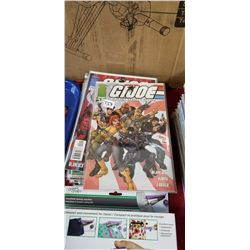59 - Shelf One
CDC Auctions
Shelf One
Bidding Has Concluded

Sold to r********r for (22.50 + 2.93) x 1 = 25.43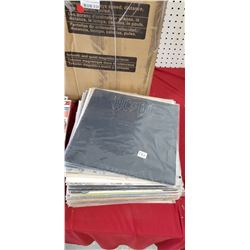60 - Shelf One
CDC Auctions
Shelf One
Bidding Has Concluded

Sold to j*****V for (15.00 + 1.95) x 1 = 16.95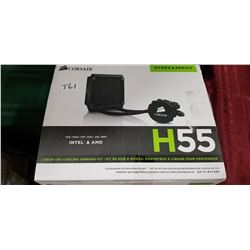61 - Shelf One
CDC Auctions
Shelf One
Bidding Has Concluded

Sold to f***r for (32.50 + 4.23) x 1 = 36.73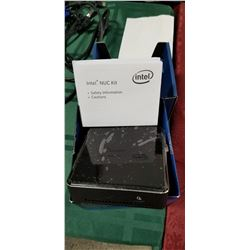64 - Intel NUC Kit
CDC Auctions
Shelf One
Bidding Has Concluded

Sold to k********z for (55.00 + 7.15) x 1 = 62.15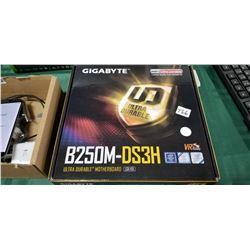66 - Shelf One
CDC Auctions
Shelf One
Bidding Has Concluded

Sold to m********O for (17.50 + 2.28) x 1 = 19.78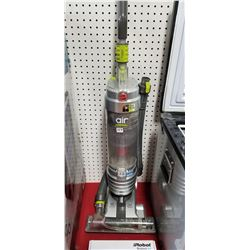69 - Shelf One
CDC Auctions
Shelf One
Bidding Has Concluded

Sold to f***r for (10.00 + 1.30) x 1 = 11.30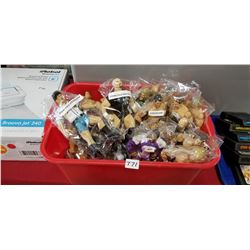71 - Shelf One
CDC Auctions
Shelf One
Bidding Has Concluded

Sold to f***r for (20.00 + 2.60) x 1 = 22.60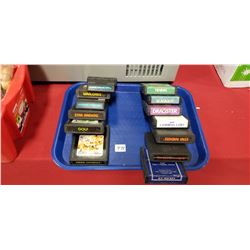72 - Shelf One
CDC Auctions
Shelf One
Bidding Has Concluded

Sold to O********c.. for (20.00 + 2.60) x 1 = 22.60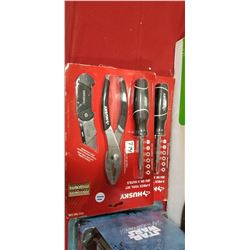74 - Shelf One
CDC Auctions
Shelf One
Bidding Has Concluded

Sold to f***r for (10.00 + 1.30) x 1 = 11.30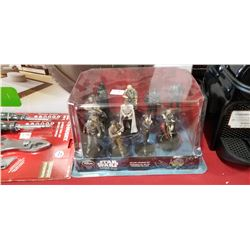75 - Shelf One
CDC Auctions
Shelf One
Bidding Has Concluded

Sold to r********r for (15.00 + 1.95) x 1 = 16.95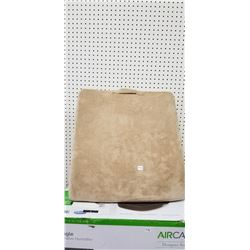77 - Shelf One
CDC Auctions
Shelf One
Bidding Has Concluded

Sold to f***r for (10.00 + 1.30) x 1 = 11.30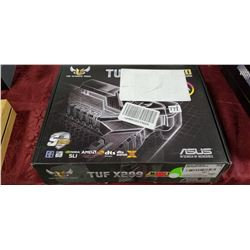78 - Shelf One
CDC Auctions
Shelf One
Bidding Has Concluded

Sold to r********r for (80.00 + 10.40) x 1 = 90.40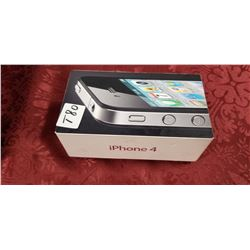80 - iPhone 4 - Used
CDC Auctions
Shelf One
Bidding Has Concluded

Sold to m********O for (27.50 + 3.58) x 1 = 31.08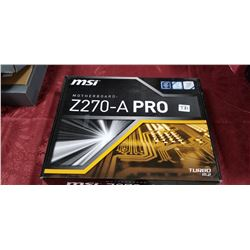81 - Shelf One
CDC Auctions
Shelf One
Bidding Has Concluded

Sold to m********O for (20.00 + 2.60) x 1 = 22.60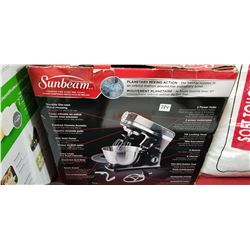84 - Shelf One
CDC Auctions
Shelf One
Bidding Has Concluded

Sold to f***r for (75.00 + 9.75) x 1 = 84.75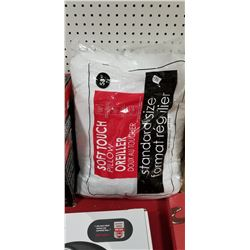86 - Shelf One
CDC Auctions
Shelf One
Bidding Has Concluded

Sold to f***r for (10.00 + 1.30) x 1 = 11.30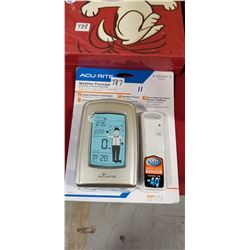87 - Shelf One
CDC Auctions
Shelf One
Bidding Has Concluded

Sold to f***r for (15.00 + 1.95) x 1 = 16.95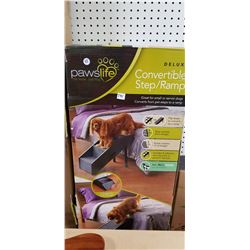91 - Shelf One
CDC Auctions
Shelf One
Bidding Has Concluded

Sold to f***r for (15.00 + 1.95) x 1 = 16.95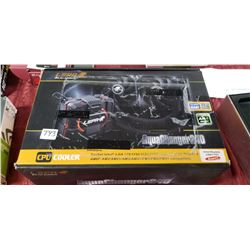93 - Shelf One
CDC Auctions
Shelf One
Bidding Has Concluded

Sold to f***r for (27.50 + 3.58) x 1 = 31.08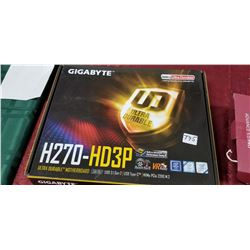95 - Shelf One
CDC Auctions
Shelf One
Bidding Has Concluded

Sold to L*********7 for (12.50 + 1.63) x 1 = 14.13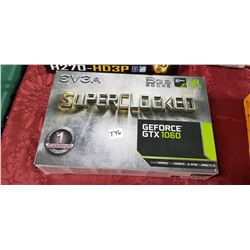96 - Shelf One
CDC Auctions
Shelf One
Bidding Has Concluded

Sold to f***r for (160.00 + 20.80) x 1 = 180.80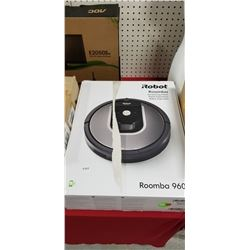99 - IRobot Roomba 960
CDC Auctions
Shelf One
Bidding Has Concluded

Sold to R*****g for (150.00 + 19.50) x 1 = 169.50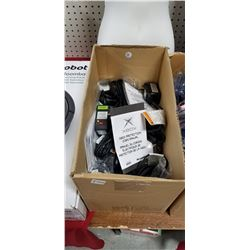100 - Shelf One
CDC Auctions
Shelf One
Bidding Has Concluded

Sold to f***r for (10.00 + 1.30) x 1 = 11.30